﻿ 大初值有界区域可压缩液晶方程组强解的爆破准则 Blow-Up Criteria for Strong Solutions of Compressible Liquid Crystal Equations in Large Initial Bounded Region

Vol. 09  No. 01 ( 2020 ), Article ID: 33939 , 14 pages
10.12677/AAM.2020.91009

Blow-Up Criteria for Strong Solutions of Compressible Liquid Crystal Equations in Large Initial Bounded Region

Licheng Qin

School of Science, Minzu University of China, Beijing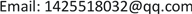Received: Dec. 25th, 2019; accepted: Jan. 7th, 2020; published: Jan. 14th, 2020ABSTRACT

Liquid crystals are widely used in real life. They are highly sensitive to light, electricity, magnetism and heat. According to this feature, various kinds of instruments with physical effects such as heat light, magnetism light, electricity light can be designed. Its research covers chemistry, physics, biology, materials science and electronics, and forms their own specialized disciplines, such as liquid crystal chemistry, liquid crystal physics, biological liquid crystal, liquid crystal optics, etc., which also arouses extensive interest of mathematicians and physicists at home and abroad. The most important problem in the liquid crystal industry is the molecular distribution law, defect, phase change phenomenon and its dynamics law observed in the liquid crystal. These problems are directly related to the manufacture of liquid crystal equipment, and the appropriate mathematical model is the most powerful tool to describe these phenomena. In view of the problems concerned in reality, from the mathematical point of view, we are concerned with the mathematical mechanism of phase transition, the global existence and regularity of solutions of dynamic equations and the blow-up criteria of strong solutions. In this paper, we consider the Cauchy problem of one-dimensional fully compressible liquid crystal equations without heat conduction. In the case of a large initial value bounded region, we use the method of precise energy estimation and nonlinear functional analysis to prove the existence of blow-up criteria for the strong solution of this system of equations.

Keywords:One-Dimensional Liquid Crystal Equations, Completely Compressible, Blow-Up Criterion of Strong Solution1. 引言

2. 研究内容及研究方法

2.1. 研究内容

2.2. 具体研究方法

$\left\{\begin{array}{l}{\stackrel{˜}{\rho }}_{t}+{\left(\stackrel{˜}{\rho }\stackrel{˜}{u}\right)}_{x}=0\\ {\left(\stackrel{˜}{\rho }\stackrel{˜}{u}\right)}_{t}+{\left(\stackrel{˜}{\rho }{\stackrel{˜}{u}}^{2}+\stackrel{˜}{P}\right)}_{x}={\left(\mu {u}_{x}\right)}_{x}-{\stackrel{˜}{n}}_{x}{\stackrel{˜}{n}}_{xx}\\ {\stackrel{˜}{n}}_{t}+\stackrel{˜}{u}{\stackrel{˜}{n}}_{x}={\stackrel{˜}{n}}_{xx}+{|{\stackrel{˜}{n}}_{x}|}^{2}\stackrel{˜}{n}\\ {\stackrel{˜}{P}}_{t}+\stackrel{˜}{u}{\stackrel{˜}{P}}_{x}+\gamma \stackrel{˜}{P}{\stackrel{˜}{u}}_{x}=\left(\gamma -1\right)\mu {\left({\stackrel{˜}{u}}_{x}\right)}^{2}+\left(\gamma -1\right){|{\stackrel{˜}{n}}_{xx}+{|{\stackrel{˜}{n}}_{x}|}^{2}\stackrel{˜}{n}|}^{2}\end{array}$ (*)

$\stackrel{˜}{P}:\left[0,+\infty \right)\to R$ 是局部Lipschitz连续函数 (2.1.1)

${\left(\stackrel{˜}{\rho },\stackrel{˜}{u},\stackrel{˜}{n},\stackrel{˜}{P}\right)|}_{t=0}=\left({\stackrel{˜}{\rho }}_{\text{0}},{\stackrel{˜}{u}}_{0},{\stackrel{˜}{n}}_{0},{\stackrel{˜}{P}}_{0}\right)$，其中 ${\stackrel{˜}{\rho }}_{\text{0}}$ 恒为常数。 (2.1.2)

Ω是有界光滑区域，且

${\left(\stackrel{˜}{u},\frac{\partial \stackrel{˜}{n}}{\partial v}\right)|}_{\partial \Omega }=0$ (2.1.3)

${\int }_{\text{0}}^{{T}_{\ast }}\left({‖{\stackrel{˜}{u}}_{x}‖}_{{L}^{\infty }}+{‖{\stackrel{˜}{n}}_{x}‖}_{{L}^{\infty }}^{2}\right)\text{d}t=+\infty$ (2.1.4)

${\int }_{\text{0}}^{{T}_{\ast }}\left({‖{\stackrel{˜}{u}}_{x}‖}_{{L}^{\infty }}+{‖{\stackrel{˜}{n}}_{x}‖}_{{L}^{\infty }}^{2}\right)\text{d}t<+\infty$ (2.1.5)

$\underset{0\le t<{T}_{*}}{\mathrm{sup}}{‖{\stackrel{˜}{n}}_{x}‖}_{{L}^{r}}^{r}+{\int }_{0}^{{T}_{*}}{\int }_{\Omega }{|{\stackrel{˜}{n}}_{x}|}^{r-2}{|{\stackrel{˜}{n}}_{xx}|}^{2}\text{d}x\text{d}t\le C$ (2.2.1)

${\stackrel{˜}{n}}_{tx}-{\stackrel{˜}{n}}_{xxx}=-{\left(u{\stackrel{˜}{n}}_{x}\right)}_{x}+{\left({|{\stackrel{˜}{n}}_{x}|}^{2}\stackrel{˜}{n}\right)}_{x}$ (2.2.2)

$\begin{array}{l}\frac{\text{d}}{\text{d}t}\int {|{\stackrel{˜}{n}}_{x}|}^{r}\text{d}x+r\left(r-1\right)\int {|{\stackrel{˜}{n}}_{x}|}^{r-2}{|{\stackrel{˜}{n}}_{xx}|}^{2}\text{d}x\\ =r\int {\left({|{\stackrel{˜}{n}}_{x}|}^{2}\stackrel{˜}{n}\right)}_{x}{|{\stackrel{˜}{n}}_{x}|}^{r-2}{\stackrel{˜}{n}}_{x}\text{d}x-r\int {\left(u{\stackrel{˜}{n}}_{x}\right)}_{x}{|{\stackrel{˜}{n}}_{x}|}^{r-2}{\stackrel{˜}{n}}_{x}\text{d}x={I}_{1}+{I}_{2}\end{array}$ (2.2.3)

${\left({|{\stackrel{˜}{n}}_{x}|}^{2}\stackrel{˜}{n}\right)}_{x}={|{\stackrel{˜}{n}}_{x}|}^{2}{\stackrel{˜}{n}}_{x}+{\left({|{\stackrel{˜}{n}}_{x}|}^{2}\right)}_{x}\stackrel{˜}{n}$，且 ${\stackrel{˜}{n}}_{x}\stackrel{˜}{n}=0$

${I}_{1}=r\int {|{\stackrel{˜}{n}}_{x}|}^{r+2}\text{d}x\le {‖{\stackrel{˜}{n}}_{x}‖}_{{L}^{\infty }}^{2}\int {|{\stackrel{˜}{n}}_{x}|}^{r}\text{d}x$

$\begin{array}{c}{I}_{2}=-r\int {\left(\stackrel{˜}{u}{\stackrel{˜}{n}}_{x}\right)}_{x}{|{\stackrel{˜}{n}}_{x}|}^{r-2}{\stackrel{˜}{n}}_{x}\text{d}x\\ =-r\int \left(\stackrel{˜}{u}{\stackrel{˜}{n}}_{xx}{|{\stackrel{˜}{n}}_{x}|}^{r-2}{\stackrel{˜}{n}}_{x}+{\stackrel{˜}{u}}_{x}{\stackrel{˜}{n}}_{x}{|{\stackrel{˜}{n}}_{x}|}^{r-2}{\stackrel{˜}{n}}_{x}\right)\text{d}x\\ =-\int \stackrel{˜}{u}{\left({|{\stackrel{˜}{n}}_{x}|}^{r}\right)}_{x}\text{d}x-r\int {\stackrel{˜}{u}}_{x}{|{\stackrel{˜}{n}}_{x}|}^{r}\text{d}x\\ =\left(1-r\right)\int {\stackrel{˜}{u}}_{x}{|{\stackrel{˜}{n}}_{x}|}^{r}\text{d}x\\ \le {‖{\stackrel{˜}{u}}_{x}‖}_{{L}^{\infty }}\int {|{\stackrel{˜}{n}}_{x}|}^{r}\text{d}x\end{array}$

${I}_{1},{I}_{2}$ 代入(2.2.3)式整理得：

$\begin{array}{l}\frac{\text{d}}{\text{d}t}\int {|{\stackrel{˜}{n}}_{x}|}^{r}\text{d}x+r\left(r-1\right)\int {|{\stackrel{˜}{n}}_{x}|}^{r-2}{|{\stackrel{˜}{n}}_{xx}|}^{2}\text{d}x\\ \le \left({‖{\stackrel{˜}{n}}_{x}‖}_{{L}^{\infty }}^{2}+{‖{\stackrel{˜}{u}}_{x}‖}_{{L}^{\infty }}\right)\int {|{\stackrel{˜}{n}}_{x}|}^{r}\text{d}x\end{array}$

$\begin{array}{l}\int {|{\stackrel{˜}{n}}_{x}|}^{r}\text{d}x+r\left(r-1\right){\int }_{0}^{t}{\int }_{\Omega }{|{\stackrel{˜}{n}}_{x}|}^{r-2}{|{\stackrel{˜}{n}}_{xx}|}^{2}\text{d}x\text{d}s\\ \le \int {|{\left({\stackrel{˜}{n}}_{0}\right)}_{x}|}^{r}\text{d}x\cdot \mathrm{exp}\left({\int }_{0}^{{T}_{*}}\left({‖{\stackrel{˜}{n}}_{x}‖}_{{L}^{\infty }}^{2}+{‖{\stackrel{˜}{u}}_{x}‖}_{{L}^{\infty }}\right)\text{d}t\right)\\ \le C\end{array}$

$\underset{0\le t<{T}_{*}}{\mathrm{sup}}{‖{\stackrel{˜}{n}}_{xx}‖}_{{L}^{2}}^{2}+{\int }_{0}^{{T}_{*}}{\int }_{\Omega }{|{\stackrel{˜}{n}}_{tx}|}^{2}\text{d}x\text{d}t\le C$ (2.3.1)

$\begin{array}{l}\frac{\text{d}}{\text{d}t}\int {|{\stackrel{˜}{n}}_{xx}|}^{2}\text{d}x+\int {|{\stackrel{˜}{n}}_{tx}|}^{2}\text{d}x=\int \left({\left({|{\stackrel{˜}{n}}_{x}|}^{2}\stackrel{˜}{n}\right)}_{x}-{\left(\stackrel{˜}{u}{\stackrel{˜}{n}}_{x}\right)}_{x}\right){\stackrel{˜}{n}}_{tx}\text{d}x\\ \le C\left(\epsilon \right)\int \left({|{\stackrel{˜}{n}}_{x}|}^{6}+{|{\stackrel{˜}{u}}_{x}|}^{2}{|{\stackrel{˜}{n}}_{x}|}^{2}+{|\stackrel{˜}{u}|}^{2}{|{\stackrel{˜}{n}}_{xx}|}^{2}+{|{\stackrel{˜}{n}}_{x}|}^{2}{|{\stackrel{˜}{n}}_{xx}|}^{2}\right)\text{d}x+\epsilon {‖{\stackrel{˜}{n}}_{tx}‖}_{{L}^{2}}^{2}\\ \le \epsilon {‖{\stackrel{˜}{n}}_{tx}‖}_{{L}^{2}}^{2}+C+C{‖{\stackrel{˜}{n}}_{x}‖}_{{L}^{\infty }}^{2}\left({‖{\stackrel{˜}{u}}_{x}‖}_{{L}^{2}}^{2}+{‖{\stackrel{˜}{n}}_{xx}‖}_{{L}^{2}}^{2}\right)+C\int {|\stackrel{˜}{u}|}^{2}{|{\stackrel{˜}{n}}_{xx}|}^{2}\text{d}x\end{array}$ (2.3.2)

$\begin{array}{c}{\int |\stackrel{˜}{u}|}^{2}{|{\stackrel{˜}{n}}_{xx}|}^{2}\text{d}x\le {‖\stackrel{˜}{u}‖}_{{L}^{6}}^{2}{‖{\stackrel{˜}{n}}_{xx}‖}_{{L}^{3}}^{2}\le {‖{\stackrel{˜}{u}}_{x}‖}_{{L}^{2}}^{2}{‖{\stackrel{˜}{n}}_{x}‖}_{{L}^{6}}{‖{\stackrel{˜}{n}}_{xxx}‖}_{{L}^{2}}\\ \le {‖{\stackrel{˜}{u}}_{x}‖}_{{L}^{2}}^{2}{‖{\stackrel{˜}{n}}_{xxx}‖}_{{L}^{2}}\le C\left(\epsilon \right){‖{\stackrel{˜}{u}}_{x}‖}_{{L}^{2}}^{4}+\epsilon {‖{\stackrel{˜}{n}}_{xxx}‖}_{{L}^{2}}^{2}\end{array}$ (2.3.3)(2.3.4)

${‖{\stackrel{˜}{n}}_{xxx}‖}_{{L}^{2}}^{2}\le {‖{\stackrel{˜}{n}}_{tx}‖}_{{L}^{2}}^{2}+{‖{\stackrel{˜}{n}}_{x}‖}_{{L}^{\infty }}^{2}\left({‖{\stackrel{˜}{u}}_{x}‖}_{{L}^{2}}^{2}+{‖{\stackrel{˜}{n}}_{xx}‖}_{{L}^{2}}^{2}\right)+{‖{\stackrel{˜}{u}}_{x}‖}_{{L}^{2}}^{4}+C$ (2.3.5)

$\int {|u|}^{2}{|{\stackrel{˜}{n}}_{xx}|}^{2}\text{d}x\le C+\epsilon {‖{\stackrel{˜}{n}}_{tx}‖}_{{L}^{2}}^{2}+{‖{\stackrel{˜}{n}}_{x}‖}_{{L}^{\infty }}^{2}\left({‖{\stackrel{˜}{u}}_{x}‖}_{{L}^{2}}^{2}+{‖{\stackrel{˜}{n}}_{xx}‖}_{{L}^{2}}^{2}\right)+{‖{u}_{x}‖}_{{L}^{2}}^{4}$ (2.3.6)

$\begin{array}{c}\frac{\text{d}}{\text{d}t}\int {|{\stackrel{˜}{n}}_{xx}|}^{2}\text{d}x+\int {|{\stackrel{˜}{n}}_{tx}|}^{2}\text{d}x\le C+{‖{\stackrel{˜}{u}}_{x}‖}_{{L}^{2}}^{4}+{‖{\stackrel{˜}{n}}_{x}‖}_{{L}^{\infty }}^{2}{‖{\stackrel{˜}{u}}_{x}‖}_{{L}^{2}}^{2}+{‖{\stackrel{˜}{n}}_{x}‖}_{{L}^{\infty }}^{2}{‖{\stackrel{˜}{n}}_{xx}‖}_{{L}^{2}}^{2}\\ \le C+{‖{\stackrel{˜}{n}}_{x}‖}_{{L}^{\infty }}^{2}{‖{\stackrel{˜}{n}}_{xx}‖}_{{L}^{2}}^{2}\end{array}$ (2.3.7)

$\begin{array}{l}\int {|{\stackrel{˜}{n}}_{xx}|}^{2}\text{d}x+{\int }_{\text{0}}^{t}{\int }_{\Omega }{|{\stackrel{˜}{n}}_{tx}|}^{2}\text{d}x\text{d}s\\ \le CT\cdot \mathrm{exp}\left(CT\right)+\int {|{\left({\stackrel{˜}{n}}_{0}\right)}_{xx}|}^{2}\text{d}x\cdot \mathrm{exp}\left({\int }_{0}^{{T}_{*}}{‖{\stackrel{˜}{n}}_{x}‖}_{{L}^{\infty }}^{2}\text{d}t\right)\\ \le C\end{array}$ (2.3.8)

${‖{\stackrel{˜}{n}}_{xxx}‖}_{{L}^{2}}^{2}\le {‖{\stackrel{˜}{n}}_{tx}‖}_{{L}^{2}}^{2}+{‖{\stackrel{˜}{n}}_{x}‖}_{{L}^{\infty }}^{2}\left({‖{\stackrel{˜}{u}}_{x}‖}_{{L}^{2}}^{2}+{‖{\stackrel{˜}{n}}_{xx}‖}_{{L}^{2}}^{2}\right)+{‖{\stackrel{˜}{u}}_{x}‖}_{{L}^{2}}^{4}+C\le C$ (2.3.9)

${\stackrel{˜}{n}}_{xx}\in {L}_{x}^{\infty }\left(\Omega \right),\text{\hspace{0.17em}}\text{\hspace{0.17em}}{\stackrel{˜}{n}}_{x}\in {L}_{x}^{\infty }\left(\Omega \right)$ (2.3.10)

$|{\stackrel{˜}{n}}_{t}|\le \left(|\stackrel{˜}{u}||{\stackrel{˜}{n}}_{x}|+|{\stackrel{˜}{n}}_{xx}|+{|{\stackrel{˜}{n}}_{xx}|}^{2}\right)\in {L}_{t}^{\infty }{L}_{x}^{\infty }\left(\Omega ×\left[0,{T}_{*}\right]\right)$ (2.3.1)

$\left\{\begin{array}{l}{\partial }_{t}\eta \left(y,t\right)=\stackrel{˜}{u}\left(\eta \left(y,t\right),t\right)\\ \eta \left(y,0\right)=y\end{array}$

$\left\{\begin{array}{l}{J}_{t}={u}_{y}\\ {\rho }_{0}{u}_{t}-\mu {\left(\frac{{u}_{y}}{J}\right)}_{y}+{P}_{y}+{\left(\frac{{n}_{y}}{J}\right)}_{y}\frac{{n}_{y}}{J}=0\\ {n}_{t}=\frac{1}{J}{\left(\frac{{n}_{y}}{J}\right)}_{y}+{|\frac{{n}_{y}}{J}|}^{2}n\\ {P}_{t}+\gamma P\frac{{u}_{y}}{J}=\left(\gamma -1\right)\mu {\left(\frac{{u}_{y}}{J}\right)}^{\text{2}}+\left(\gamma -1\right){|\frac{1}{J}{\left(\frac{{n}_{y}}{J}\right)}_{y}+{|\frac{{n}_{y}}{J}|}^{2}n|}^{2}\end{array}$ (**)

P仍是局部Lipschitz连续函数， $\Omega$ 变为 $\partial \Omega$ 也仍是有界光滑区域，且

${\left(u,\frac{\partial n}{\partial {v}_{1}}\right)|}_{\partial {\Omega }_{1}}=0$ (3.1.1)

(2.1.5)式变为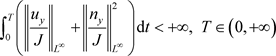(3.1.2)

3. 强解存在的定义

$\underset{y\in {\Omega }_{\text{1}},t\in \left(0,T\right)}{\mathrm{inf}}J\left(y,t\right)>0,\text{\hspace{0.17em}}\text{\hspace{0.17em}}P\ge 0\text{\hspace{0.17em}}\text{\hspace{0.17em}}\text{on}\text{ }\text{\hspace{0.17em}}{\Omega }_{\text{1}}×\left(0,T\right)$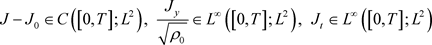$\sqrt{{\rho }_{\text{0}}}u\in C\left(\left[0,T\right];{L}^{2}\right),\text{\hspace{0.17em}}\text{\hspace{0.17em}}{u}_{y}\in {L}^{\infty }\left(\left[0,T\right];{L}^{2}\right),\text{\hspace{0.17em}}\text{\hspace{0.17em}}\left(\sqrt{{\rho }_{\text{0}}}{u}_{t},\frac{{u}_{yy}}{\sqrt{{\rho }_{\text{0}}}}\right)\in {L}^{2}\left(\left[0,T\right];{L}^{2}\right)$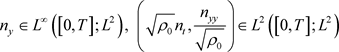$P\in C\left(\left[0,T\right];{L}^{2}\right),\text{\hspace{0.17em}}\text{\hspace{0.17em}}\frac{{P}_{y}}{\sqrt{{\rho }_{\text{0}}}}\in {L}^{\infty }\left(\left[0,T\right];{L}^{2}\right),\text{\hspace{0.17em}}\text{\hspace{0.17em}}{P}_{t}\in {L}^{4}\left(\left[0,T\right];{L}^{2}\right)$

$\underset{{\Omega }_{\text{1}}}{\int }{\left(\frac{1}{2}{\rho }_{0}{u}^{2}\right)}_{t}\text{d}y+\underset{{\Omega }_{\text{1}}}{\int }\mu \frac{{\left({u}_{y}\right)}^{2}}{J}\text{d}y-\underset{{\Omega }_{\text{1}}}{\int }P{u}_{y}\text{d}y+\underset{{\Omega }_{\text{1}}}{\int }{\left(\frac{{n}_{y}}{J}\right)}_{y}\frac{{n}_{y}}{J}u\text{d}y=0$ (3.1.3)

${\left(JP\right)}_{t}={J}_{t}P+J{P}_{t}=P{u}_{y}+J{P}_{t}$

$\underset{R}{\int }{\left(\frac{1}{2}{\rho }_{0}{u}^{2}+\frac{JP}{\gamma -1}\right)}_{t}\text{d}y-\underset{R}{\int }J{|{n}_{t}|}^{2}\text{d}y+\underset{R}{\int }{\left(\frac{{n}_{y}}{J}\right)}_{y}\frac{{n}_{y}}{J}u\text{d}y=0$ (3.1.4)

$\underset{R}{\int }J{|{n}_{t}|}^{2}\text{d}y=\underset{R}{\int }{n}_{t}{\left(\frac{{n}_{y}}{J}\right)}_{y}\text{d}y+\underset{R}{\int }J{n}_{t}n{|\frac{{n}_{y}}{J}|}^{2}$

${|\stackrel{˜}{n}|}^{2}={|n|}^{2}=|\stackrel{˜}{n}|=1$ 知， ${n}_{t}n={n}_{y}n=0$

$\underset{R}{\int }J{|{n}_{t}|}^{2}\text{d}y=-\underset{R}{\int }{\left(\frac{\frac{1}{2}{\left({n}_{y}\right)}^{2}}{J}\right)}_{t}\text{d}y+\underset{R}{\int }{\left(\frac{{n}_{y}}{J}\right)}_{y}\frac{{n}_{y}}{J}u\text{d}y$ (3.1.5)(3.1.6)

$\underset{R}{\int }\left(\frac{1}{2}{\rho }_{0}{u}^{2}+\frac{JP}{\gamma -1}+\frac{\frac{1}{2}{\left({n}_{y}\right)}^{2}}{J}\right)\text{d}y-\underset{R}{\int }\left(\frac{1}{2}{\rho }_{0}{u}_{0}^{2}+\frac{{P}_{0}}{\gamma -1}+\frac{1}{2}{\left({n}_{0}{}_{y}\right)}^{2}\right)\text{d}y=0$ (3.1.7)

${J}_{t}={u}_{y}$ 得：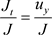，进一步有 ${|\mathrm{ln}J|}_{t}=\frac{{u}_{y}}{J}$，两边同时在 $\left(0,t\right)$ 上积分得：

$\mathrm{ln}J-\mathrm{ln}{J}_{0}={\int }_{0}^{t}\frac{{u}_{y}}{J}\text{d}t$，于是有 $J={J}_{0}{\text{e}}^{{\int }_{0}^{t}\frac{{u}_{y}}{J}\text{d}t}\le {J}_{0}{\text{e}}^{{\int }_{0}^{t}{‖\frac{{u}_{y}}{J}‖}_{{L}^{\infty }}\text{d}t}\le C$，定义J的上界为 $\stackrel{¯}{J}$

${\partial }_{t}\left({\rho }_{0}u\right)-\mu {\partial }_{ty}\left(\mathrm{ln}J\right)+{P}_{y}+{\left(\frac{{n}_{y}}{J}\right)}_{y}\frac{{n}_{y}}{J}=0$ (3.1.8)

$\begin{array}{l}{\int }_{{z}_{0}}^{z}\left({\rho }_{0}u-{\rho }_{0}{u}_{0}\right)\text{d}y-\mu \left[\mathrm{ln}J\left(z,t\right)-\mathrm{ln}J\left({z}_{0},t\right)\right]+{\int }_{0}^{t}\left[P\left(z,\tau \right)-P\left({z}_{0},\tau \right)\right]\text{d}\tau \\ +\frac{1}{2}{\int }_{0}^{t}\left[{\left(\frac{{n}_{y}\left(z,t\right)}{J\left(z,t\right)}\right)}^{2}-{\left(\frac{{n}_{y}\left({z}_{0},t\right)}{J\left({z}_{0},t\right)}\right)}^{2}\right]\text{d}\tau =0\end{array}$

${z}_{0}\to -\infty$ 得： $J\to 1,P\to 0,{n}_{y}\to 0$；于是：

${\int }_{-\infty }^{z}\left({\rho }_{0}u-{\rho }_{0}{u}_{0}\right)\text{d}y-\mu \mathrm{ln}J\left(z,t\right)+{\int }_{0}^{t}P\left(z,\tau \right)\text{d}\tau +\frac{1}{2}{\int }_{0}^{t}{\left(\frac{{n}_{y}\left(z,t\right)}{J\left(z,t\right)}\right)}^{2}\text{d}\tau =0$

$\begin{array}{c}J\left(z,t\right)\ge {\text{e}}^{\frac{1}{\mu }{\int }_{-\infty }^{z}\left({\rho }_{0}u-{\rho }_{0}{u}_{0}\right)\text{d}y+{\int }_{0}^{t}P\left(z,\tau \right)\text{d}\tau +\frac{1}{2}{\int }_{0}^{t}{\left(\frac{{n}_{y}\left(z,t\right)}{J\left(z,t\right)}\right)}^{2}\text{d}\tau }\\ \ge {\text{e}}^{\frac{1}{\mu }{\int }_{-\infty }^{z}\left({\rho }_{0}u-{\rho }_{0}{u}_{0}\right)\text{d}y}\end{array}$

$\begin{array}{l}|{\int }_{-\infty }^{z}\left({\rho }_{0}u-{\rho }_{0}{u}_{0}\right)\text{d}y|\le {\int }_{-\infty }^{z}{\rho }_{0}\left(|u|-|{u}_{\text{0}}|\right)\text{d}y\\ \le {\left(\underset{{\Omega }_{\text{1}}}{\int }{\rho }_{0}\text{d}y\right)}^{\frac{1}{2}}{\left(\underset{{\Omega }_{\text{1}}}{\int }\frac{{\rho }_{0}}{2}\left(2{|u|}^{2}-2{|{u}_{\text{0}}|}^{2}\right)\text{d}y\right)}^{\frac{1}{2}}\\ \le {‖{\rho }_{0}‖}_{1}^{\frac{1}{2}}{\left(4{E}_{0}\right)}^{\frac{1}{2}}=2{‖{\rho }_{0}‖}_{1}^{\frac{1}{2}}{E}_{0}^{\frac{1}{2}}\end{array}$

$\underset{0\le t\le T}{\mathrm{sup}}{‖G‖}_{2}^{2}+{\int }_{0}^{T}{‖\frac{{G}_{y}}{\sqrt{{\rho }_{0}}}‖}_{2}^{2}\text{d}t+{\left({\int }_{0}^{T}{‖G‖}_{\infty }^{4}\text{d}t\right)}^{\frac{1}{2}}\le C{\text{e}}^{CT}{‖{G}_{0}‖}_{2}^{2}$

${G}_{t}=\mu {\left(\frac{{u}_{y}}{J}\right)}_{t}-{P}_{t}=\frac{\mu }{J}{\left(\frac{{G}_{y}}{{\rho }_{0}}\right)}_{y}-\gamma \frac{{u}_{y}}{J}G-\frac{\mu }{J}\left(\frac{1}{{\rho }_{0}}{\left(\frac{{n}_{y}}{J}\right)}_{y}\left(\frac{{n}_{y}}{J}\right)\right)-\left(\gamma -1\right){|{n}_{t}|}^{2}$ (3.1.9)

$\begin{array}{l}\underset{{\Omega }_{\text{1}}}{\int }JG{G}_{t}\text{d}y-\mu \underset{{\Omega }_{\text{1}}}{\int }G\left(\frac{{G}_{y}}{{\rho }_{0}}\right)\text{d}y\\ =-\gamma \underset{{\Omega }_{\text{1}}}{\int }{u}_{y}{G}^{2}\text{d}y-\mu \underset{{\Omega }_{\text{1}}}{\int }G{\left(\frac{1}{{\rho }_{0}}{\left(\frac{{n}_{y}}{J}\right)}_{y}\frac{{n}_{y}}{J}\right)}_{y}\text{d}y-\left(\gamma -1\right)\underset{{\Omega }_{\text{1}}}{\int }JG{|{n}_{t}|}^{2}\text{d}y\end{array}$ (3.1.10)

$\begin{array}{l}\frac{\text{1}}{\text{2}}\frac{\text{d}}{\text{d}t}\underset{{\Omega }_{\text{1}}}{\int }J{G}^{\text{2}}\text{d}y+\mu \underset{{\Omega }_{\text{1}}}{\int }\frac{{\left({G}_{y}\right)}^{2}}{{\rho }_{0}}\text{d}y\\ =\left(\frac{\text{1}}{\text{2}}-\gamma \right)\underset{{\Omega }_{\text{1}}}{\int }{u}_{y}{G}^{2}\text{d}y-\mu \underset{{\Omega }_{\text{1}}}{\int }G{\left(\frac{1}{{\rho }_{0}}{\left(\frac{{n}_{y}}{J}\right)}_{y}\frac{{n}_{y}}{J}\right)}_{y}\text{d}y-\left(\gamma -1\right)\underset{{\Omega }_{\text{1}}}{\int }JG{|{n}_{t}|}^{2}\text{d}y\end{array}$ (3.1.11)

$\left(\frac{1}{2}-\gamma \right)\underset{{\Omega }_{\text{1}}}{\int }{u}_{y}{G}^{2}\text{d}y$ 的估计，利用Gagliardo-Nirenberg不等式和Young不等式得：

$\begin{array}{c}\left(\frac{1}{2}-\gamma \right)\underset{{\Omega }_{\text{1}}}{\int }{u}_{y}{G}^{2}\text{d}y=\left(2\gamma -1\right)\underset{{\Omega }_{\text{1}}}{\int }uG{G}_{y}\text{d}y\le C\underset{{\Omega }_{\text{1}}}{\int }|u||G||{G}_{y}|\text{d}y\\ \le C\underset{{\Omega }_{\text{1}}}{\int }\sqrt{{\rho }_{0}}|u||G||\frac{{G}_{y}}{\sqrt{{\rho }_{0}}}|\text{d}y\le C{‖\sqrt{{\rho }_{0}}u‖}_{{L}^{2}}{‖G‖}_{{L}^{\infty }}{‖\frac{{G}_{y}}{\sqrt{{\rho }_{0}}}‖}_{{L}^{2}}\\ \le C{‖\sqrt{{\rho }_{0}}u‖}_{{L}^{2}}{‖G‖}_{{L}^{2}}^{\frac{1}{2}}{‖\frac{{G}_{y}}{\sqrt{{\rho }_{0}}}‖}_{{L}^{2}}^{1}{‖\frac{{G}_{y}}{\sqrt{{\rho }_{0}}}‖}_{{L}^{2}}\\ \le C{‖G‖}_{{L}^{2}}^{\frac{1}{2}}{‖\frac{{G}_{y}}{\sqrt{{\rho }_{0}}}‖}_{{L}^{2}}^{\frac{3}{2}}\le \frac{\mu }{8}{‖\frac{{G}_{y}}{\sqrt{{\rho }_{0}}}‖}_{{L}^{2}}^{2}+C{‖G‖}_{{L}^{2}}^{2}\end{array}$ (3.1.12)

$-\mu \underset{{\Omega }_{1}}{\int }G{\left(\frac{1}{{\rho }_{0}}{\left(\frac{{n}_{y}}{J}\right)}_{y}\frac{{n}_{y}}{J}\right)}_{y}\text{d}y$ 的估计：

$\begin{array}{l}-\mu \underset{R}{\int }G{\left(\frac{1}{{\rho }_{0}}{\left(\frac{{n}_{y}}{J}\right)}_{y}\frac{{n}_{y}}{J}\right)}_{y}\text{d}y=\mu \underset{R}{\int }\frac{{G}_{y}}{\sqrt{{\rho }_{0}}}\frac{1}{\sqrt{{\rho }_{0}}}{\left(\frac{{n}_{y}}{J}\right)}_{y}\frac{{n}_{y}}{J}\text{d}y\\ \le \frac{\mu }{16}{‖\frac{{G}_{y}}{\sqrt{{\rho }_{0}}}‖}_{{L}^{2}}^{2}+C{‖\frac{\text{1}}{\sqrt{{\rho }_{0}}}‖}_{{L}^{2}}^{2}{‖\frac{{n}_{y}}{J}‖}_{{L}^{\infty }}^{2}{‖{\left(\frac{{n}_{y}}{J}\right)}_{y}‖}_{{L}^{2}}^{2}=\frac{\mu }{16}{‖\frac{{G}_{y}}{\sqrt{{\rho }_{0}}}‖}_{{L}^{2}}^{2}+C\end{array}$ (3.1.13)

${n}_{t}={\stackrel{˜}{n}}_{t}+\stackrel{˜}{u}{\stackrel{˜}{n}}_{x}$${\stackrel{˜}{n}}_{t},{\stackrel{˜}{n}}_{x},\stackrel{˜}{u}\in {L}_{t}^{\infty }{L}_{x}^{\infty }\left(\Omega ×\left[0,{T}_{\ast }\right]\right)$，J有上下界，于是有 ${‖{n}_{t}‖}_{{L}^{2}\left({\Omega }_{1}\right)}^{2}\le C$

$-\left(\gamma -1\right)\underset{R}{\int }JG{|{n}_{t}|}^{2}\text{d}y$ 的估计，利用Gagliardo-Nirenberg不等式和Young不等式得：

$\begin{array}{c}-\left(\gamma -1\right)\underset{{\Omega }_{1}}{\int }JG{|{n}_{t}|}^{2}\text{d}y\le \left(\gamma -1\right)\underset{{\Omega }_{1}}{\int }\stackrel{¯}{J}|G|{|{n}_{t}|}^{2}\text{d}y\le \left(\gamma -1\right)\stackrel{¯}{J}{‖G‖}_{{L}^{\infty }}{‖{n}_{t}‖}_{{L}^{2}}^{2}\le \left(\gamma -1\right)\stackrel{¯}{J}{‖{n}_{t}‖}_{{L}^{2}}^{2}{‖G‖}_{{L}^{2}}^{\frac{1}{2}}{‖\frac{{G}_{y}}{\sqrt{{\rho }_{0}}}‖}_{{L}^{2}}^{\frac{1}{2}}\\ \le C{\left(\left(\gamma -1\right)\stackrel{¯}{J}{‖{n}_{t}‖}_{{L}^{2}}^{2}\right)}^{2}+C{\left({‖G‖}_{{L}^{2}}^{\frac{1}{2}}{‖\frac{{G}_{y}}{\sqrt{{\rho }_{0}}}‖}_{{L}^{2}}^{\frac{1}{2}}\right)}^{2}\le C+C{‖G‖}_{{L}^{2}}^{2}+\frac{\mu }{16}{‖\frac{{G}_{y}}{\sqrt{{\rho }_{0}}}‖}_{{L}^{2}}^{2}\end{array}$ (3.1.14)

$\frac{\text{d}}{\text{d}t}\underset{R}{\int }J{G}^{\text{2}}\text{d}y+\frac{3\mu }{2}{‖\frac{{G}_{y}}{\sqrt{{\rho }_{0}}}‖}_{{L}^{2}}^{2}\le C{‖G‖}_{{L}^{2}}^{2}+C$ (3.1.15)

${‖G‖}_{{L}^{2}}^{2}\left(t\right)+{\int }_{\text{0}}^{t}{‖\frac{{G}_{y}}{\sqrt{{\rho }_{0}}}‖}_{{L}^{2}}^{2}\text{d}\tau \le C\left({\int }_{0}^{t}{‖G‖}_{{L}^{2}}^{2}\text{d}\tau +{‖{G}_{0}‖}_{{L}^{2}}^{2}\right)+CT$ (3.1.16)

$\underset{0\le t\le T}{\mathrm{sup}}{‖G‖}_{{L}^{2}}^{2}+{\int }_{0}^{T}{‖\frac{{G}_{y}}{\sqrt{{\rho }_{0}}}‖}_{{L}^{2}}^{2}\text{d}t\le C{\text{e}}^{CT}{‖{G}_{0}‖}_{{L}^{2}}^{2}+{C}_{2}T$ (3.1.17)

${\int }_{0}^{T}{‖G‖}_{{L}^{\infty }}^{4}\text{d}t\le {\int }_{0}^{T}{‖G‖}_{{L}^{2}}^{2}{‖{G}_{y}‖}_{{L}^{2}}^{2}\text{d}t\le C\left(T\right)$ (3.1.18)${\int }_{0}^{T}\left({‖{P}_{t}‖}_{2}^{4}+{‖\left(\sqrt{{\rho }_{0}}{u}_{t},\frac{{u}_{yy}}{\sqrt{{\rho }_{0}}}\right)‖}_{2}^{2}\right)\text{d}t\le C$

$\begin{array}{c}{P}_{t}+\mu {\left(\frac{{u}_{y}}{J}\right)}^{2}=\gamma \mu {\left(\frac{{u}_{y}}{J}\right)}^{2}-\gamma \frac{{u}_{y}}{J}P+\left(\gamma -1\right){|{n}_{t}|}^{2}\\ =\gamma \frac{{u}_{y}}{J}G+\left(\gamma -1\right){|{n}_{t}|}^{2}\\ \le \mu {\left(\frac{{u}_{y}}{J}\right)}^{2}+\frac{{\gamma }^{2}}{4\mu }{G}^{2}+\left(\gamma -1\right){|{n}_{t}|}^{2}\end{array}$

${P}_{t}\le \frac{{\gamma }^{2}}{4\mu }{G}^{2}+\left(\gamma -1\right){|{n}_{t}|}^{2}$ (3.1.19)

$P\le {P}_{0}+\frac{{\gamma }^{2}}{4\mu }{\int }_{0}^{t}{G}^{2}\text{d}t+\left(\gamma -1\right){\int }_{0}^{t}{|{n}_{t}|}^{2}\text{d}t$

${n}_{t}={\stackrel{˜}{n}}_{t}+\stackrel{˜}{u}{\stackrel{˜}{n}}_{x}$${\stackrel{˜}{n}}_{t},{\stackrel{˜}{n}}_{x},\stackrel{˜}{u}\in {L}_{t}^{\infty }{L}_{x}^{\infty }\left(\Omega ×\left[0,{T}_{\ast }\right]\right)$，J有上下界，于是有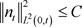${‖P‖}_{q}\le {‖{P}_{0}+C‖}_{q}+\frac{{\gamma }^{2}}{4\mu }{\int }_{0}^{t}{‖G‖}_{2q}^{2}\text{d}t$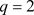时：

${‖P‖}_{2}\le {‖{P}_{0}+C‖}_{2}+\frac{{\gamma }^{2}}{4\mu }{\int }_{0}^{t}{‖G‖}_{4}^{2}\text{d}t\le C$ (3.1.20)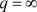时：

${‖P‖}_{2}\le {‖{P}_{0}+C‖}_{\infty }+\frac{{\gamma }^{2}}{4\mu }{\int }_{0}^{t}{‖G‖}_{\infty }^{2}\text{d}t\le C$ (3.1.21)

$\underset{0\le t\le T}{\mathrm{sup}}\left({‖P‖}_{2}+{‖P‖}_{\infty }\right)\le C$ (3.1.22)

${P}_{t}+\frac{\text{1}}{\mu }{\left(P+\frac{2-\gamma }{2}G\right)}^{2}=\frac{{\gamma }^{\text{2}}}{4\mu }{G}^{2}+\left(\gamma -1\right){|{n}_{t}|}^{2}$ (3.1.23)

$\begin{array}{c}\frac{\text{d}}{\text{d}t}{‖\frac{{P}_{y}}{\sqrt{{\rho }_{0}}}‖}_{2}^{2}\le C\left({‖P‖}_{{L}^{\infty }}+{‖G‖}_{{L}^{\infty }}\right)\left({‖\frac{{P}_{y}}{\sqrt{{\rho }_{0}}}‖}_{{L}^{2}}+{‖\frac{{G}_{y}}{\sqrt{{\rho }_{0}}}‖}_{{L}^{2}}\right){‖\frac{{P}_{y}}{\sqrt{{\rho }_{0}}}‖}_{{L}^{2}}+{‖\frac{{\partial }_{y}{|{n}_{t}|}^{2}}{\sqrt{{\rho }_{0}}}‖}_{{L}^{2}}{‖\frac{{P}_{y}}{\sqrt{{\rho }_{0}}}‖}_{{L}^{2}}\\ \le {C}_{1}+{C}_{2}{‖\frac{{\partial }_{y}{|{n}_{t}|}^{2}}{\sqrt{{\rho }_{0}}}‖}_{{L}^{2}}^{2}+{C}_{3}{‖\frac{{P}_{y}}{\sqrt{{\rho }_{0}}}‖}_{{L}^{2}}^{2}\end{array}$

${‖\frac{{\partial }_{y}{|{n}_{t}|}^{2}}{\sqrt{{\rho }_{0}}}‖}_{{L}^{2}}^{2}={\int }_{{\Omega }_{1}}\frac{4{\left({n}_{t}\right)}^{2}{\left({n}_{ty}\right)}^{2}}{{\rho }_{0}}\text{d}y\le \frac{4}{{\rho }_{0}}{‖{n}_{t}‖}_{{L}^{\infty }}^{2}{‖{n}_{ty}‖}_{{L}^{2}}^{2}$

${n}_{t}={\stackrel{˜}{n}}_{t}+\stackrel{˜}{u}{\stackrel{˜}{n}}_{x}$${\stackrel{˜}{n}}_{t},{\stackrel{˜}{n}}_{x},\stackrel{˜}{u}\in {L}_{t}^{\infty }{L}_{x}^{\infty }\left(\Omega ×\left[0,{T}_{\ast }\right]\right)$，J有上下界，于是有 ${‖{n}_{t}‖}_{{L}^{\infty }\left({\Omega }_{\text{1}}\right)}^{2}\le C$

${\stackrel{˜}{n}}_{t}={n}_{t}-\stackrel{˜}{u}{\stackrel{˜}{n}}_{x}$${\stackrel{˜}{n}}_{tx}=\frac{1}{J}{\left({n}_{t}-\stackrel{˜}{u}{\stackrel{˜}{n}}_{x}\right)}_{y}=\frac{{n}_{ty}}{J}-\frac{{u}_{y}}{J}\frac{{n}_{y}}{J}-\frac{u}{J}{\left(\frac{{n}_{y}}{J}\right)}_{y}=\frac{{n}_{ty}}{J}-{\stackrel{˜}{u}}_{x}{\stackrel{˜}{n}}_{x}-\stackrel{˜}{u}{\stackrel{˜}{n}}_{xx}$

$\frac{\text{d}}{\text{d}t}{‖\frac{{P}_{y}}{\sqrt{{\rho }_{0}}}‖}_{2}^{2}\le {C}_{\text{4}}+{C}_{\text{3}}{‖\frac{{P}_{y}}{\sqrt{{\rho }_{0}}}‖}_{{L}^{2}}^{2}$

$\underset{0\le t\le T}{\mathrm{sup}}{‖\frac{{P}_{y}}{\sqrt{{\rho }_{0}}}‖}_{2}^{2}\le CT{\text{e}}^{CT}+{‖\frac{{{P}^{\prime }}_{0}}{\sqrt{{\rho }_{0}}}‖}_{2}{\text{e}}^{CT}\le C$ (3.1.24)

${J}_{t}=\frac{J}{\mu }\left(G+P\right)$ (3.1.25)

$\begin{array}{l}{‖J-{J}_{0}‖}_{2}+{‖{J}_{t}‖}_{2}={‖{\int }_{0}^{t}{J}_{t}\text{d}\tau ‖}_{2}+{‖{J}_{t}‖}_{2}\\ \le \frac{2\stackrel{¯}{J}}{\mu }\left({\int }_{0}^{t}\left({‖G‖}_{2}+{‖P‖}_{2}\right)\text{d}\tau +{‖G‖}_{2}+{‖P‖}_{2}\right)\end{array}$

$\underset{0\le t\le T}{\mathrm{sup}}\left({‖J-{J}_{0}‖}_{2}+{‖{J}_{t}‖}_{2}\right)=C\left(\gamma ,\mu ,\stackrel{¯}{\rho },\underset{_}{J},\stackrel{¯}{J},{‖{G}_{0}‖}_{2},{‖{P}_{0}‖}_{2}\right)$ (3.1.26)

$J\left(y,t\right)=\mathrm{exp}\left\{\frac{1}{\mu }{\int }_{0}^{t}\left(G+P\right)\text{d}\tau \right\}{J}_{0}\left(y\right)$

${J}_{y}=\left(\frac{1}{\mu }{\int }_{0}^{t}\left({G}_{y}+{P}_{y}\right)\text{d}\tau {J}_{0}+{{J}^{\prime }}_{0}\right)\mathrm{exp}\left\{\frac{1}{\mu }{\int }_{0}^{t}\left(G+P\right)\text{d}\tau \right\}$

$\underset{0\le t\le T}{\text{sup}}{‖\frac{{J}_{y}}{\sqrt{{\rho }_{0}}}‖}_{{L}^{2}}\le \left(\frac{\stackrel{¯}{J}}{\mu }{\int }_{0}^{T}{‖\left(\frac{{G}_{y}}{\sqrt{{\rho }_{0}}}+\frac{{P}_{y}}{\sqrt{{\rho }_{0}}}\right)‖}_{{L}^{2}}\text{d}t+{‖\frac{{{J}^{\prime }}_{\text{0}}}{\sqrt{{\rho }_{0}}}‖}_{{L}^{2}}\right)\mathrm{exp}\left\{\frac{1}{\mu }{\int }_{0}^{T}{‖\left(G+P\right)‖}_{{L}^{\infty }}\text{d}t\right\}\le C$ (3.1.27)

$\underset{0\le t\le T}{\mathrm{sup}}{‖{u}_{y}‖}_{{L}^{2}}^{2}=\underset{0\le t\le T}{\mathrm{sup}}{‖\frac{J}{\mu }\left(G+P\right)‖}_{{L}^{2}}^{2}\le C\left(\gamma ,\mu ,\stackrel{¯}{\rho },\underset{_}{J},\stackrel{¯}{J},{‖{G}_{0}‖}_{2},{‖{P}_{0}‖}_{2}\right)$ (3.1.28)

$\begin{array}{l}{‖\frac{\text{1}}{\sqrt{{\rho }_{\text{0}}}}{\left(\frac{{n}_{y}}{J}\right)}_{y}\frac{{n}_{y}}{J}‖}_{{L}^{2}}^{2}={\int }_{{\Omega }_{\text{1}}}{\left(\frac{\text{1}}{\sqrt{{\rho }_{\text{0}}}}{\left(\frac{{n}_{y}}{J}\right)}_{y}\frac{{n}_{y}}{J}\right)}^{2}\text{d}y\\ \le \frac{\text{1}}{{\rho }_{0}}{‖\frac{{n}_{y}}{J}‖}_{{L}^{\infty }}^{2}{‖{\left(\frac{{n}_{y}}{J}\right)}_{y}‖}_{{L}^{2}}=\frac{\text{1}}{{\rho }_{0}}{‖\frac{{n}_{y}}{J}‖}_{{L}^{\infty }}^{2}{‖{\left(\frac{{n}_{y}}{J}\right)}_{y}\frac{J}{J}‖}_{{L}^{2}}\\ \le \frac{\text{1}}{{\rho }_{0}}{‖\frac{{n}_{y}}{J}‖}_{{L}^{\infty }}^{2}{‖{\left(\frac{{n}_{y}}{J}\right)}_{y}\frac{\stackrel{¯}{J}}{J}‖}_{{L}^{2}}=\frac{\stackrel{¯}{J}}{{\rho }_{0}}{‖\frac{{n}_{y}}{J}‖}_{{L}^{\infty }}^{2}{‖{\left(\frac{{n}_{y}}{J}\right)}_{y}\frac{1}{J}‖}_{{L}^{2}}\end{array}$

${\int }_{0}^{T}{‖\sqrt{{\rho }_{0}}{u}_{t}‖}_{{L}^{2}}^{2}\text{d}t\le {\int }_{0}^{T}{‖\frac{{G}_{y}}{\sqrt{{\rho }_{0}}}‖}_{{L}^{2}}^{2}\text{d}t+{\int }_{0}^{T}{‖\frac{\text{1}}{\sqrt{{\rho }_{\text{0}}}}{\left(\frac{{n}_{y}}{J}\right)}_{y}\frac{{n}_{y}}{J}‖}_{{L}^{2}}^{2}\text{d}t\le C\left(\gamma ,\mu ,\stackrel{¯}{\rho },\underset{_}{J},\stackrel{¯}{J},{‖{G}_{0}‖}_{{L}^{2}}\right)$ (3.1.29)

$\begin{array}{c}\underset{0\le t\le T}{\mathrm{sup}}{‖\sqrt{{\rho }_{\text{0}}}u‖}_{{L}^{2}\left({\Omega }_{\text{1}}\right)}=\underset{0\le t\le T}{\mathrm{sup}}{‖\sqrt{{\rho }_{\text{0}}}{u}_{0}+{\int }_{0}^{t}\sqrt{{\rho }_{\text{0}}}{u}_{t}\text{d}\tau ‖}_{{L}^{2}\left({\Omega }_{\text{1}}\right)}^{2}\\ \le {‖\sqrt{{\rho }_{\text{0}}}{u}_{0}‖}_{{L}^{2}\left({\Omega }_{\text{1}}\right)}^{2}+{\int }_{0}^{T}{‖\sqrt{{\rho }_{\text{0}}}{u}_{t}‖}_{{L}^{2}\left({\Omega }_{\text{1}}\right)}\text{d}\tau \\ \le {‖\sqrt{{\rho }_{\text{0}}}{u}_{0}‖}_{{L}^{2}\left({\Omega }_{\text{1}}\right)}^{2}+C\left(\gamma ,\mu ,\stackrel{¯}{\rho },\underset{_}{J},\stackrel{¯}{J},{‖{G}_{0}‖}_{{L}^{2}}\right)\end{array}$

${u}_{yy}={\left[\frac{J}{\mu }\left(G+P\right)\right]}_{y}=\frac{{J}_{y}}{\mu }\left(G+P\right)+\frac{J}{\mu }\left({G}_{y}+{P}_{y}\right)$

${\int }_{0}^{{T}_{\text{0}}}{‖\frac{{u}_{yy}}{\sqrt{{\rho }_{0}}}‖}_{{L}^{2}}^{2}\text{d}t\le C{\int }_{0}^{{T}_{0}}\left({‖\left(\frac{{G}_{y}}{\sqrt{{\rho }_{0}}},\frac{{P}_{y}}{\sqrt{{\rho }_{0}}}\right)‖}_{2}^{2}+{‖\left(G,P\right)‖}_{\infty }^{2}{‖\frac{{J}_{y}}{\sqrt{{\rho }_{0}}}‖}_{2}^{2}\right)\text{d}t\le C$ (3.1.30)

${\int }_{0}^{T}{‖\frac{{u}_{yy}}{\sqrt{{\rho }_{0}}}‖}_{{L}^{2}}^{2}\text{d}t\le {\int }_{0}^{T}\left({‖\left(\frac{{G}_{y}}{\sqrt{{\rho }_{0}}},\frac{{P}_{y}}{\sqrt{{\rho }_{0}}}\right)‖}_{{L}^{2}}^{2}+{‖\left(G,P\right)‖}_{{L}^{\infty }}^{2}\right)\text{d}t\le C$

${\stackrel{˜}{n}}_{xx}\in {L}^{\infty }\left(\Omega \right)$，J有上下界知 ${\left(\frac{{n}_{y}}{J}\right)}_{y}\frac{1}{J}\in {L}^{\infty }\left({\Omega }_{1}\right)$，又 ${\left(\frac{{n}_{y}}{J}\right)}_{y}\frac{1}{J}=\frac{{n}_{yy}}{{J}^{2}}-\frac{{n}_{y}{J}_{y}}{{J}^{3}}$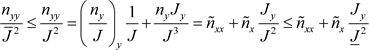${n}_{yy}\in {L}^{2}\left(\Omega 1\right)$

4. 结论

Blow-Up Criteria for Strong Solutions of Compressible Liquid Crystal Equations in Large Initial Bounded Region[J]. 应用数学进展, 2020, 09(01): 72-85. https://doi.org/10.12677/AAM.2020.91009

1. 1. Jiu, Q., Li, M. and Ye, Y. (2014) Global Classical Solution of the Cauchy Problem to 1D Compressible Navier-Stokes Equations with Large Initial Data. Journal of Differential Equations, 257, 311-350.
https://doi.org/10.1016/j.jde.2014.03.014

2. 2. Xin, Z.P. (1998) Blowup of Smooth Solutions to the Compressible Navier-Stokes Equation with Compact Density. Communications on Pure and Applied Mathematics, 51, 229-240.
3.0.CO;2-C>https://doi.org/10.1002/(SICI)1097-0312(199803)51:3<229::AID-CPA1>3.0.CO;2-C

3. 3. Huang, T., Wang, C.Y. and Wen, H.Y. (2012) Blow Up Criterion for Compressible Nematic Liquid Crystal Flows in Dimension Three. Archive for Rational Mechanics and Analysis, 204, 285-311.
https://doi.org/10.1007/s00205-011-0476-1

4. 4. Li, J.K. and Xin, Z.P. (2020) Entropy Bounded Solutions to the One-Dimensional Compressible Navier-Stokes Equations with Zero Heat Conduction and Far Field Vacuum. Advances in Mathematics, 361.
https://doi.org/10.1016/j.aim.2019.106923